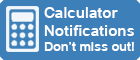Calculator Library
Free Online CalculatorsHome » 87 Calculators » Other » Fraction Calculator

Huge Domain Name Sale | Free Domains
Domain names on sale at crazy prices. Register a new domain for FREE when you buy another service.

## FRACTION CALCULATOR

INSTRUCTIONS

You can use this calculator to perform a range of fraction calculations. It will add, subtract, multiply or divide two fractions. Optionally, you can choose to reduce the result to the lowest common denominator.

1. Enter the numerator and denominator values for each of your 2 fractions.
2. To enter a whole number, use 1 as the denominator. For example, to enter 3 use 31.
3. Use the +  v dropdown setting to choose the operation — either addition, subtraction, multiplication or division.
4. Check the box if you want to reduce the result to the lowest common denominator.
5. Click the = button to see your result.

ENTER DATA HERE

 + - x ÷ Reduce to lowest common denominator

#### NOTES

1. The numerator is the top value of a fraction and denominator is the bottom. For example, in the fraction 23 the numerator is 2 and the denominator is 3. This means 2 divided by 3 and represents 2 pieces of a whole divided into 3 equally sized pieces.
2. The lowest common denominator is the smallest common multiple for the denominators of two or more fractions. For example, the fractions 46 and 69 have a lowest common denominator of 3 and can both be reduced to 23, which has the same value.
3. Fractions can also be shown as a decimal or percentage. For example, 310 is the same value as 0.3 or 30%.
4. Percentages represent a fraction of 100. For example, 30% represents 30100 or 30 divided by 100.
5. The reciprocal of a fraction swaps the numerator and denominator. For example, the reciprocal of 23 is 32. If you multiply a fraction by its reciprocal, the result will always equal 1.
6. Fractions can be classed as proper or improper. If the numerator and denominator are both positive, a proper fraction has a numerator less than the denominator. Otherwise, the fraction is improper because the result includes an integer value. For example, 54 is an improper fraction because it should be expressed as 114.